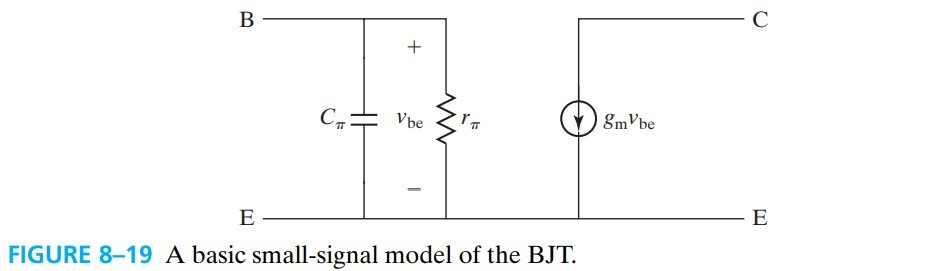# Bipolar Transistors

\begin{align*} \newcommand{\dif}{\mathop{}\!\mathrm{d}} \newcommand{\belowarrow}{\mathop{#1}\limits_{\uparrow}} \newcommand{\bd}{\boldsymbol} \newcommand{\L}{\mathscr{L}} \end{align*}

# 8.1 Introduction to the BJT

bipolar junction transistor or BJT 是 1948 年由贝尔实验室发明的。尽管现在 IC 里面大部分都是低功耗的 MOS 管，但由于 BJT 具有高速、低噪、高输出功率的优点，所以一些放大器还是用 BJT.

BJT 的结构：

• NPN
• heavily doped N+ emitter 射极
• P-type base 基极
• N-type collector 集电极
• PNP
• heavily doped P+ emitter 射极
• N-type base 基极
• P-type collector 集电极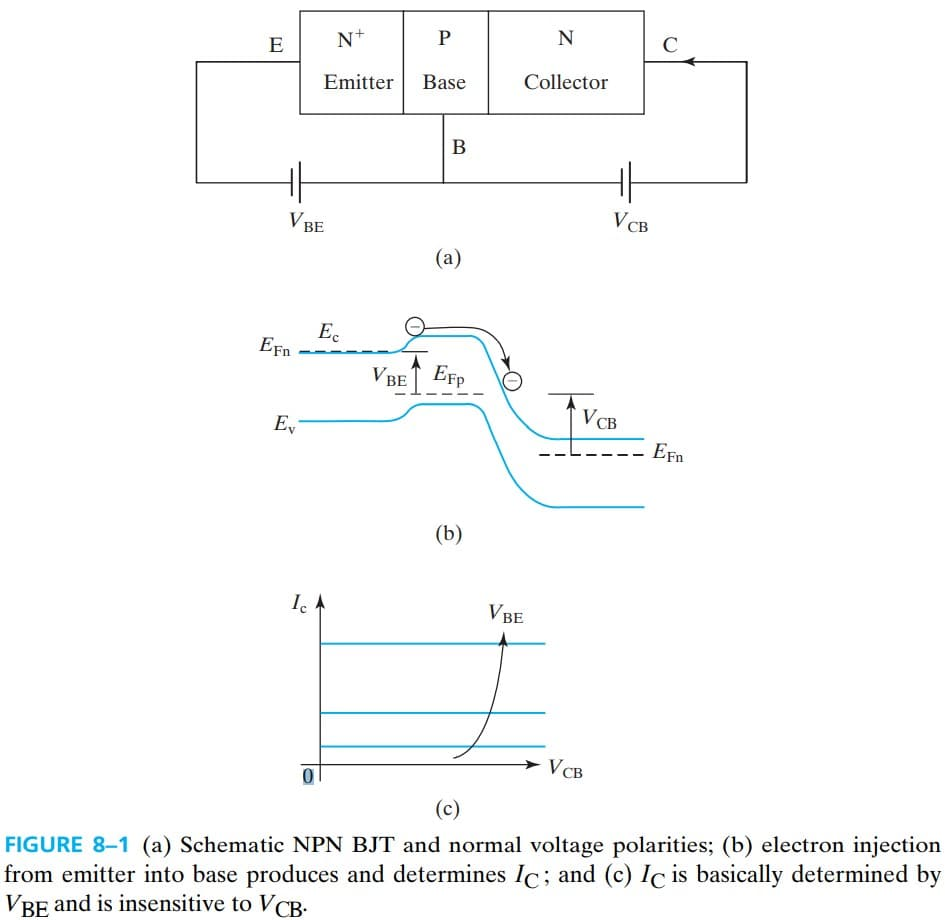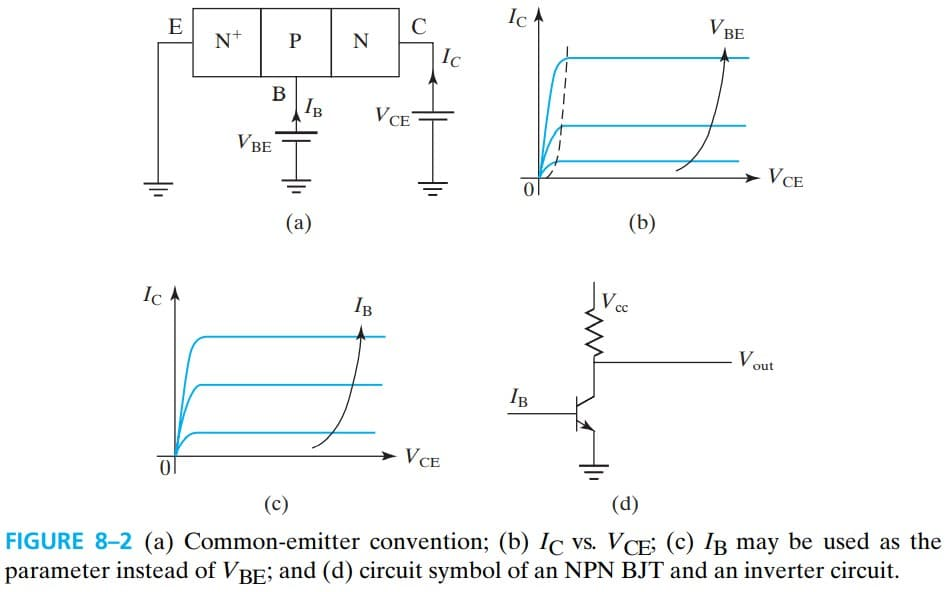# 8.2 Collector Current

\begin{aligned} I_C &= A_E J_n = \left|A_E q D_B \frac{\dif n}{\dif x}\right|\\ \end{aligned}

$\frac{\dif^2 n'}{\dif x^2} = \frac{n'}{L_B^2}\\ 其中，L_B \equiv \sqrt{D_B \tau_B}$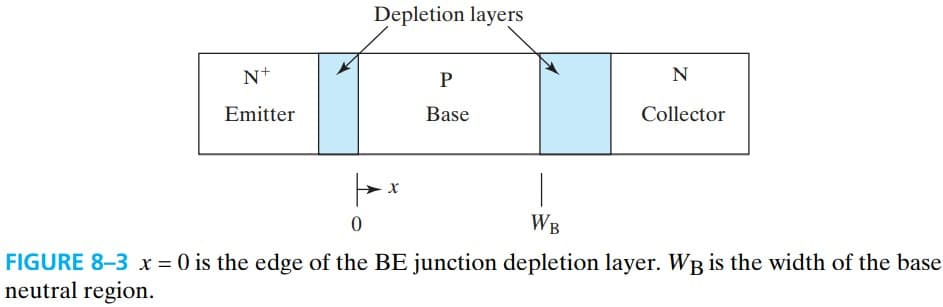$正偏 n'(0) = n_{B0} (e^{qV_{BE}/KT}-1) \\ 反偏 n'(W_B) = n_{B0} (e^{qV_{BC}/KT}-1) \approx -n_{B0} \approx 0$

$n_{B0}$ 是原本的少子浓度，即 $n_{B0}=n_i^2 / N_B$，$N_B$ 是 base doping concentration.

$n'(x)=n_{B0} (e^{q V_{BE}/kT}-1) \dfrac{\sinh \left(\dfrac{W_B-x}{L_B}\right)}{\sinh (W_B/L_B)}$

$n'(x) = n'(0)\frac{W_B-x}{W_B}\\ = \frac{n_{iB}^2}{N_B} (e^{q V_{BE}/kT}-1) \left( 1 - \frac{x}{W_B}\right) \tag{8.2.6}$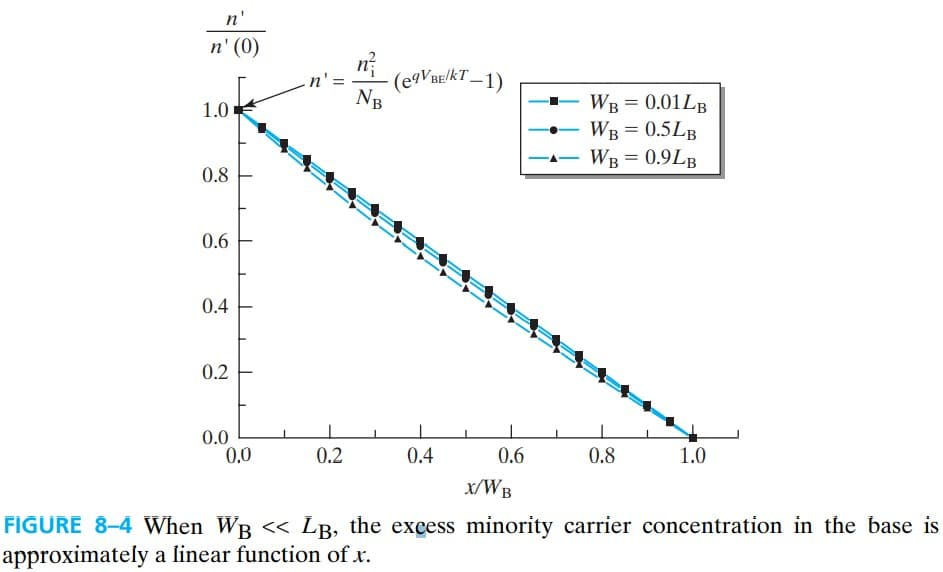\begin{aligned} I_C &= A_E J_n = \left|A_E q D_B \frac{\dif n}{\dif x}\right|\\ &= A_E q D_B \frac{n'(0)}{W_B} （代入上面的解）\\ &= A_E q \frac{D_B}{W_B} \frac{n_{iB}^2}{N_B} (e^{q V_{BE}/kT}-1) \end{aligned} \tag{8.2.7}

$A_E$ 是 BJT 的面积，准确来说是射级的面积（就是少子注入的面积），$n_{iB}$ 是基区材料的 intrinsic carrier concentration

$G_B = \frac{n_i^2}{n_{iB}^2} \frac{N_B}{D_B} W_B = \frac{n_i^2}{n_{iB}^2} \frac{p}{D_B} W_B \tag{8.2.10}$

$G_B = \int_0^{W_B} \frac{n_i^2}{n_{iB}^2} \frac{p}{D_B} \dif x \tag{8.2.11}$

\begin{aligned} I_C &= A_E \frac{q n_i^2}{G_B} (e^{q V_{BE}/kT}-1)\\ &= I_S (e^{q V_{BE}/kT}-1) \end{aligned}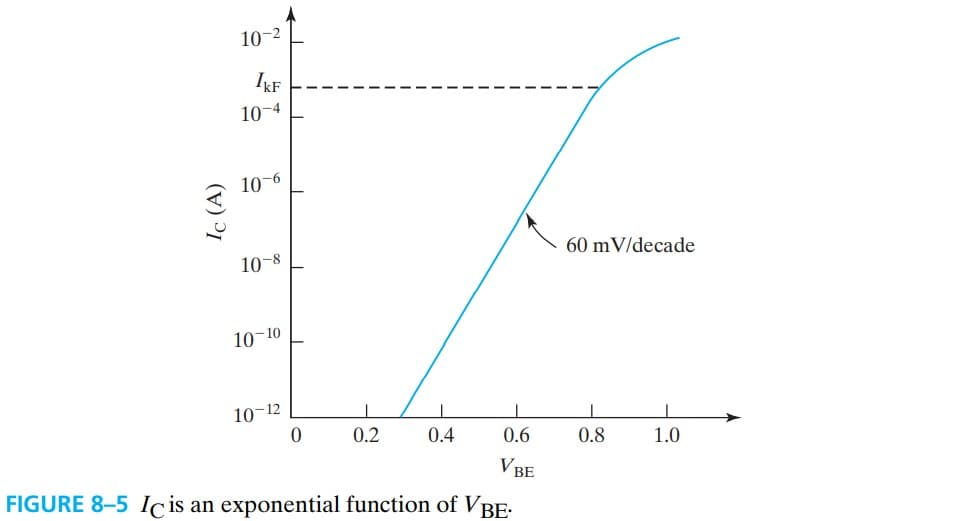$G_B$ 基本上包含了所有可能影响 $I_C$ 的内部因素：

• $n_{iB}$ 材料
• $D_B$
• $N_B$ 或 $p$ 参杂浓度

## 8.2.1 High-Level Injection Effect

$n' = p' \gg N_B$

$n \approx p$

$n \approx p \approx n_i e ^{qV_{BE}/2kT}$

$G_B \propto p = n_i e ^{qV_{BE}/2kT}$

$I_C \propto n_i e ^{qV_{BE}/2kT} \tag{8.2.17}$

# 8.3 Base Current

Emitter 发射的电子要到 base，base 发射的空穴要到 emitter，这两个是类似的（但不是完全对称，注意前面都是 $A_E$），所以上面推导的公式可以同样用来求 $I_B$

$I_B = A_E \frac{q n_i^2}{G_E} (e^{q V_{BE}/kT}-1) \tag{8.3.1}\\ G_E = \int_0^{W_E} \frac{n_i^2}{n_{iE}^2}\frac{n}{D_E} \dif x\\ n=n(x)$

$G_E$ 是 emitter Gummel number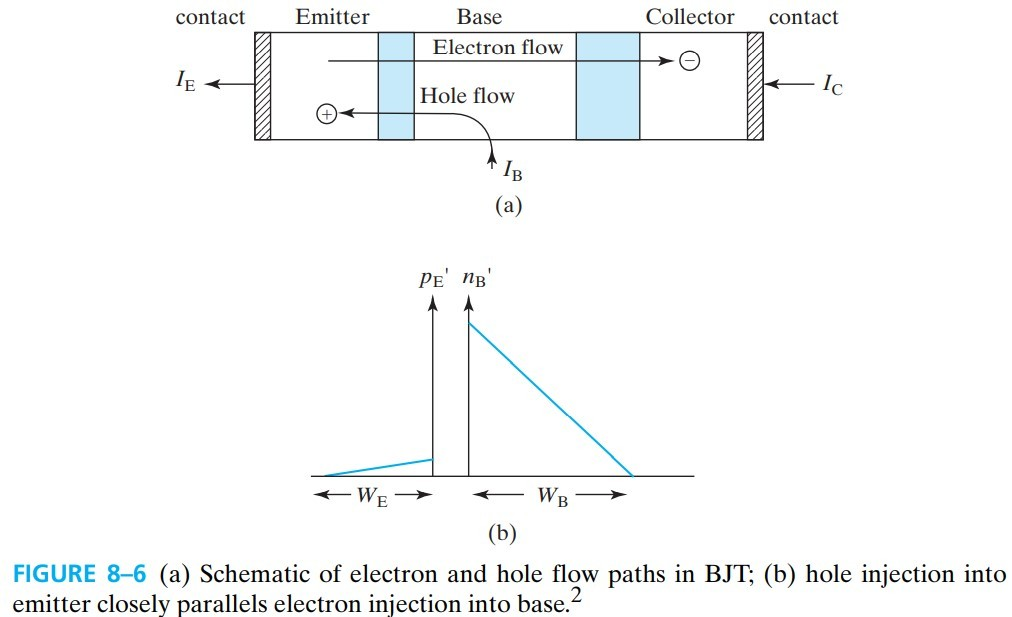# 8.4 Current Gain

• common-emitter current gain: $\beta_F \equiv \dfrac{I_C}{I_B} \tag{8.4.1}$
• common-base current gain: $\alpha_F = \dfrac{I_C}{I_E}=\dfrac{I_C}{I_B+I_C}$

$\alpha_F =\dfrac{I_C}{I_B+I_C}=\dfrac{I_C/I_B}{1+I_C/I_B}=\frac{\beta_F}{1+\beta_F} \tag{8.4.3}$ $\beta_F = \frac{I_C}{I_E-I_C}=\frac{I_C/I_E}{1-I_C/I_E}=\frac{\alpha_F}{1-\alpha_F} \tag{8.4.4}$

$\alpha_F$ 大约是 0.99（接近于1），根据上面的关系式，$\beta_F$ 是一个很大的值（100以上）。

$\beta_F = \frac{G_E}{G_B}=\frac{D_B W_E N_E n_{iB}^2}{D_E W_B N_B n_{iE}^2}$

$D$ 和 $W$ 的取值范围有限，影响不大，主要是 $N_B$ 和 $N_E$ 起作用，所以 emitter 要重掺杂 $N_E \gg N_B$

$\gamma_E = \frac{I_C}{I_E}=\frac{I_E-I_B}{I_E}=\frac{I_C}{I_C+I_B}=\frac{1}{1+G_B/G_E}$

## 8.4.1 Emitter Bnad Gap Narrowing

$n_{iE}^2 = n_i^2 e^{\Delta E_{gE}/kT}$

## 8.4.2 Gummel Plot and βF Fall-Off at High and Low $I_C$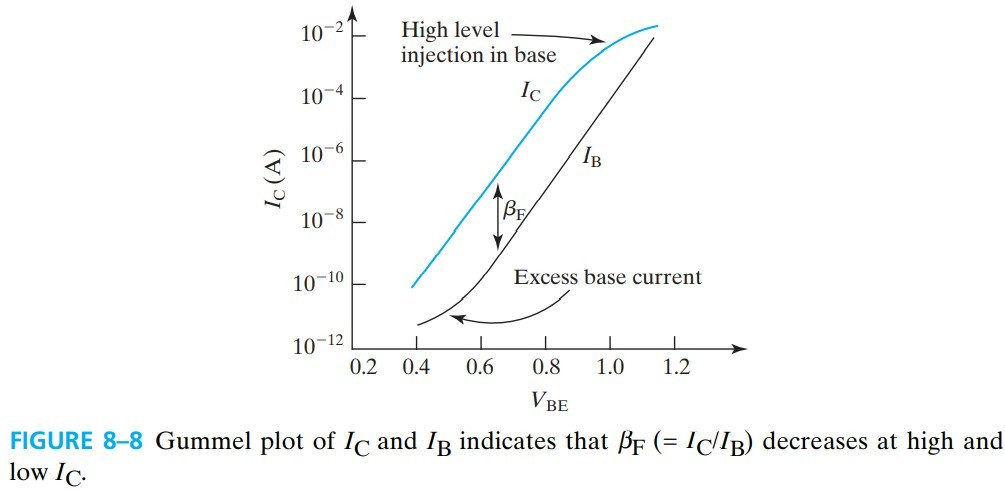$\beta_F$ 在 $I_C$ 很大或很小时都会下降。

# 8.5 Base-Width Modulation by Collector Voltage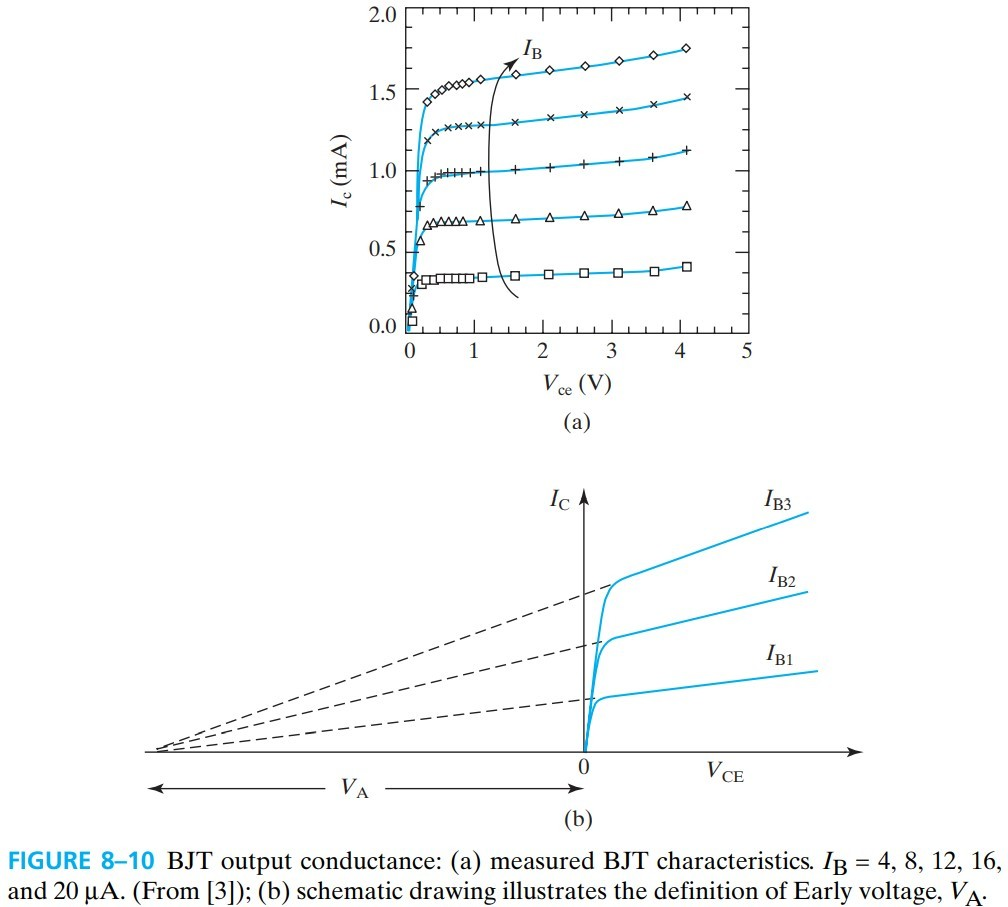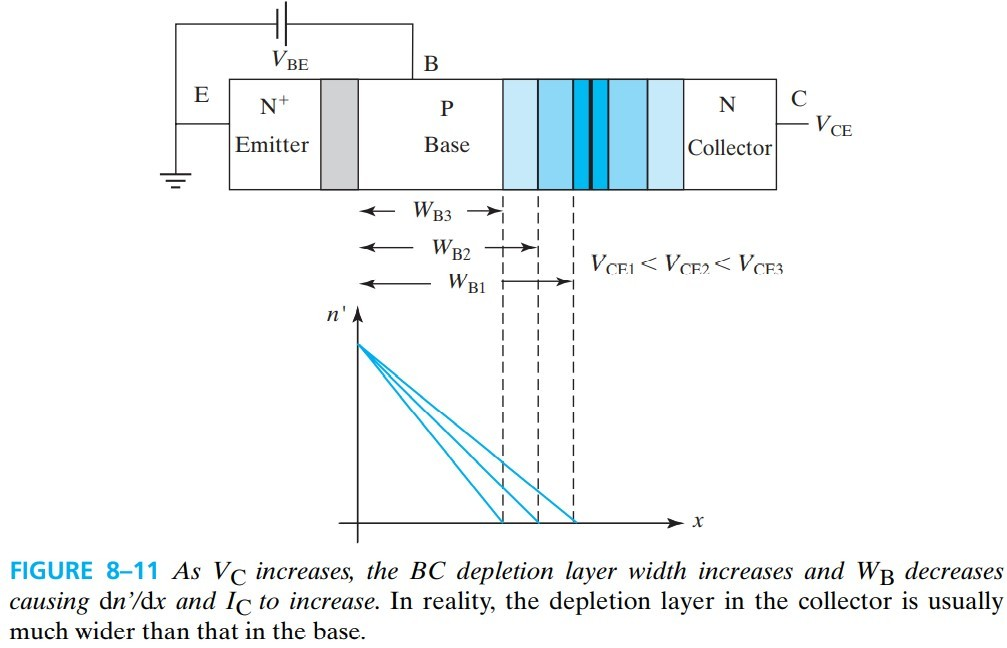$r_0 \equiv \left( \frac{\partial I_C }{ \partial V_{CE}} \right)^{-1} = \frac{V_A}{I_C} \tag{8.5.1}$

1. increase the base width
2. increase the base doping concentration, $N_B$, or
3. decrease the collector doping concentration, $N_C$.

# 8.7 Transit Time and Charge Storage

$\tau_F \equiv \frac{Q_F}{I_C}$

## 8.7.1 Base Charge Storage and Base Transit Time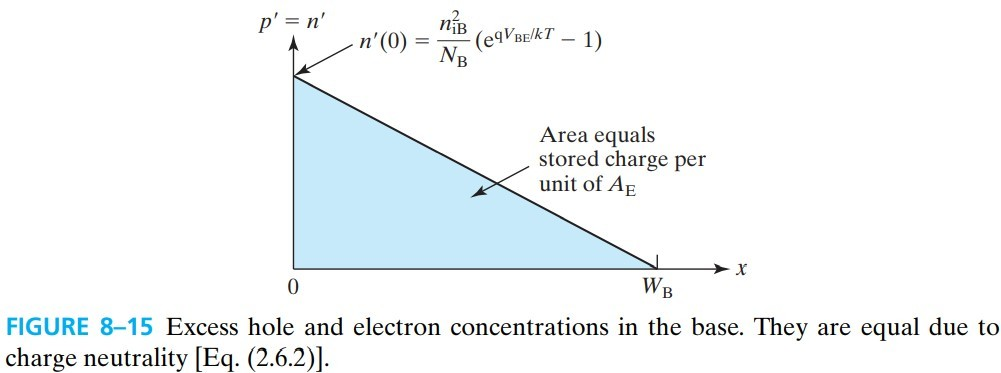$Q_{FB} = q A_E \cdot n'(0) \cdot W_B / 2$

$\frac{Q_{FB}}{I_C} \equiv \tau_{FB} = \frac{W_B^2}{2 D_B}$

$\tau_{FB}$ 就是 base transit time. 可以看出，要提高速度，必须要减小 $\tau_{FB}$，也就是要减小 $W_B$

## 8.7.3 Drift Transistor-Built-In Base Field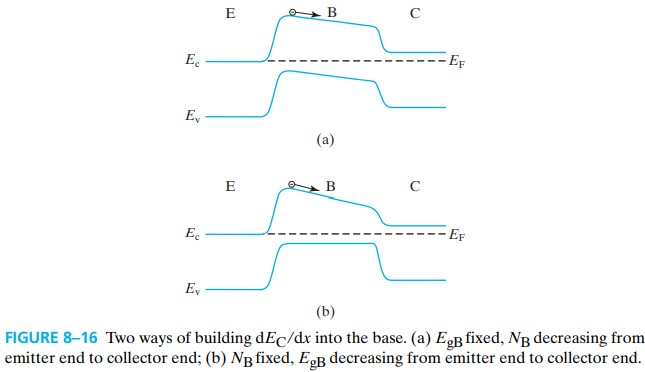# 8.8 Small-Signal Model

$I_C = I_S e^{q V_{BE}/kT}$

$g_m \equiv \frac{\dif I_C}{\dif V_{BE}}=\frac{q}{kT} I_S e^{q V_{BE}/ kT}=I_C/\frac{kT}{q}$

$\frac{1}{r_\pi} = \frac{\dif I_B}{\dif V_{BE}} = \frac{1}{\beta_F} \frac{\dif I_C}{\dif V_{BE}}=\frac{g_m}{\beta_F}\\ r_\pi = \beta_F / g_m$ $C_\pi = \frac{\dif Q_F}{\dif V_{BE}}=\frac{\dif}{\dif V_{BE}} \tau_F I_C=\tau_F g_m$